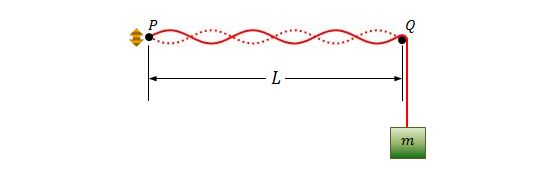Classical Mechanics

# Waves - Problem SolvingYou are watching a marching band practice outdoors. Near you are two trumpet players, one marching directly towards you and one away from you at the same speed. Each trumpet player is playing an A (440 Hz) and you hear a beat between the two sounds at a frequency of 2 Hz. How fast are the trumpet players marching in m/s?

Details and assumptions

• The speed of sound is $$340~\mbox{m/s}$$.
• The trumpet players aren't moving very fast.

A wire with mass $$100\text{ g}$$ and length $$10.00\text{ m}$$ is held on the two points $$P$$ and $$Q$$ under a tension of $$240\text{ N}.$$ Pulse $$1$$ is sent along the wire from $$P$$ at time $$t=0,$$ and pulse $$2$$ is sent along the wire from $$Q$$ at time $$t=35.0\text{ ms}.$$ Approximately, at what distance $$x$$ from $$P$$ do the pulses begin to meet?A string, which is fixed at point $$P,$$ is stretched by a block with mass $$m$$ at point $$Q,$$ as shown in the above figure. Point $$P$$ is generating a sinusoidal wave but the amplitude of $$P$$ is small enough to be considered as a node. The distance between $$P$$ and $$Q$$ is $$L=1.50\text{ m},$$ and the linear density of the string is $$\mu=1.60\text{ g/m}.$$ If the oscillating frequency of $$P$$ is $$f=130\text{ Hz}$$ and is setting up sixth harmonic on the string, what is the approximate mass $$m$$ of the block?

Assumptions and Details

• The gravitational acceleration is $$g=9.80\text{ m/s}^2.$$

If the linear densities of the heaviest and lightest steel wires of a certain piano are $$3.1 \text{ g/m}$$ and $$0.26\text{ g/m},$$ respectively, what is the approximate ratio of the diameter of the two wires?

What is the approximate speed of a transverse wave in a rope of length $$1.75\text{ m}$$ and mass $$60.0\text{ g}$$ under a tension of $$700\text{ N}?$$

×

Problem Loading...

Note Loading...

Set Loading...